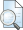## ANALISIS AUTOKORELASI DAN PENGARUHNYA TERHADAP MODEL REGRESI LINIER SEDERHANA

IKA WULANSARI, RETNO (2011) ANALISIS AUTOKORELASI DAN PENGARUHNYA TERHADAP MODEL REGRESI LINIER SEDERHANA. Other thesis, University of Muhammadiyah Malang.

Full text not available from this repository.

## Abstract

In searching for the regression model performed an assessment. Estimation of parameters of simple linear regression model has assumptions that must be met. One of these assumptions is deviate, there will be auto correlation. Auto correlation is a deviation assumption that there is no correlation between the disturbance variables in a certain period with the previous time period. When there is auto correlation, the regression model of influence, so we need to know how to cope with the occurrence of auto correlation. Assessment of a simple linear regression model using OLS method to note the assumptions that must be met. The result OLS estimator should have the properties of linear, unbiased and minimum variance. This is stated in the theorem of Gauss-Marcov. The importance of this trait seen when applied in a standard significance test (standard) against and , and create confidence interval estimates. After that need to find a coefficient of determination is used to measure the level of accuracy the best from the regression analysis. Effect of auto correlation in the model will lead to results far from the original estimation, so that the estimated model no longer efficient or not meaningful. To overcome the auto correlation of which needs to be done is to look for auto correlation coefficients, and then transform the model and applying OLS to perform the fitting again. Therefore, the model used is simple linear regression model with the OLS estimation, it can also be searched on multiple linear regression model and can also be done with other methods to estimating parameters of the model.

Item Type: Thesis (Other) Q Science > QA Mathematics Faculty of Teacher Training and Education > Department of Mathematics Education (84202) Gusti Vani Putri Cahya 29 Jan 2015 03:17 29 Jan 2015 03:17 http://eprints.umm.ac.id/id/eprint/15352

### Actions (login required)View Item